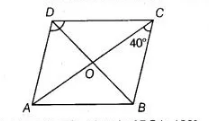# A B C D is a rhombus such that`
Question:

$A B C D$ is a rhombus such that $\angle A C B=40^{\circ}$, then $\angle A D B$ is

(a) $40^{\circ}$

(b) $45^{\circ}$

(c) $50^{\circ}$

(d) $60^{\circ}$

Solution:

(c) Given, $A B C D$ is a rhombus such that $\angle A C B=40^{\circ} \Rightarrow \angle O C B=40^{\circ}$

Since, $A D \| B C$

$\angle D A C=\angle B C A=40^{\circ}$ [alternate interior angles]

Also, $\angle A O D=90^{\circ}$

[diagonals of a rhombus are perpendicular to each other]We know that, sum of all angles of a triangle $A D O$ is $180^{\circ}$.

$\therefore \quad \angle A D O+\angle D O A+\angle O A D=180^{\circ}$

$\angle A D O=180^{\circ}-\left(40^{\circ}+90^{\circ}\right)$

$=180^{\circ}-130^{\circ}=50^{\circ}$

$\Rightarrow \quad \angle A D B=50^{\circ}$